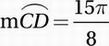# SAT Math Multiple Choice Question 718: Answer and Explanation

### Test Information

Question: 718

13.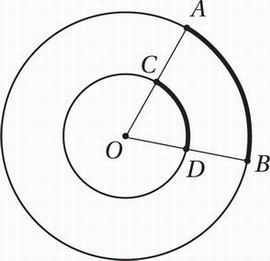Note: Figure not drawn to scale.

The figure above shows two concentric circles with center O. If OD = 3, DB = 5, and the length of arc AB is 5π, what is the length of arc CD?

• A.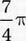• B.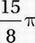• C. 3π
• D.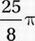Explanation:

B

Special Topics (arcs) HARD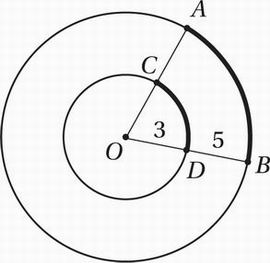First, we should make sure we mark up the diagram with the measurements we know: OD = 3 and DB = 5. This means that the radius of the small circle is 3 and the radius of the large circle is 8. Notice that sectors AOB and COD share a central angle, and therefore are similar. So the measures of arc CD and arc AB are

in a ratio of 3:8.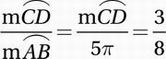Cross multiply: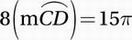Divide by 8: